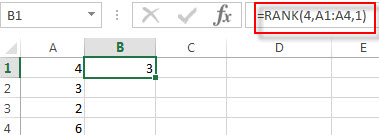# Excel RANK Function

This post will guide you how to use the RANK function with syntax and examples in Microsoft excel.

### Description

The Excel RANK function returns the rank of a given number in a supplied range of cells.  If there are duplicated values in a list of values, it will be set the same rank. This function has been replaced by the RANK.EQ function in Excel 2010.

The RANK function is a build-in function in Microsoft Excel and it is categorized as a Statistical Function.

The RANK function is available in Excel 2016, Excel 2013, Excel 2010, Excel 2007, Excel 2003, Excel XP, Excel 2000, Excel 2011 for Mac.

### Syntax

The syntax of the RANK function is as below:

`= RANK (number,ref,[order])`

Where the RANK function arguments are:

• Number – This is a required argument.  The number for which you want to get the rank.
• ref – This is a required argument. An array or range of cells, or cell reference that contain the supplied number.
• Order – This is an optional argument. . A number specifying how to rank number. If order is 0 or omitted, the RANK function will rank the numbers in descending order. If the order is not equal to 0, the RANK function will rank numbers in ascending order.

### Excel RANK Function Examples

The below examples will show you how to use Excel RANK Function to get the rank of a given value within a supplied range of cells.

Example 1:  to get the rank of 4 in a given range of cells (A1:A4), using the following formula:

`=RANK(5,A1:A4,1)`### More Excel Rank Function Examples

• Rank Data with Multiple Criteria
Assuming that you want to rank data against multiple criteria for a range of cells in your worksheet. How to achieve it. You need to create a new complex formula based on the SUMPRODUCT function. .…
•  Rank values in a column based a specific value in another column
Assuming that you have a list of data contains two columns and the first column is product list and another is Sales number. You want to rank the sales number of a specified product name. You can try to write a complex formula based on the IF function and the COUNTIFS function to achieve the result..…
• Reverse Rank Order
Assuming that you have a formula based on RANK function that ranks numbers by biggest to smallest by default. But you want to go the opposite from the smallest to biggest.…
• Rank data Based on the Number of Occurrences
You need to get the number of occurrences for the selected range of cells, then rank those number using the RANK function…

Related Posts

Sort/Rank Numeric Values with Duplicate Values Exist

Excel built-in RANK function can sort a set of values. If there are duplicate numbers, then the rank number is also duplicated. See the following example: There are two numbers “100” in range A2:A9, they are both the third largest ...

How to Rank Numbers without Repetitive Ranks in Excel

This post will guide you how to rank range numbers with unique ranking in Excel. How do I use Rank function to get the unique ranking of values for a range with duplicate values in Excel 2013/ 2016. Ranking without ...

Rank data Based on the Number of Occurrences

This post will guide you how to rank data by the number of occurrences in excel. How do I rand cells by the number of occurrences with formula in excel. Rand Data by Occurrences Assuming that you have a list ...

Reverse Rank Order in Excel

This post will guide you how to rank data in reverse order in Excel. You can use the RANK function to rank data in excel, and the biggest value will be ranked as 1 by default. How to reverse this ...

Sort Dynamic Data

This post will guide you how to sort the dynamic data automatically in your worksheet. How do I sort dynamic range of data with a formula in Excel. Sort Dynamic Data Assuming that you have a list of data in ...

Sidebar